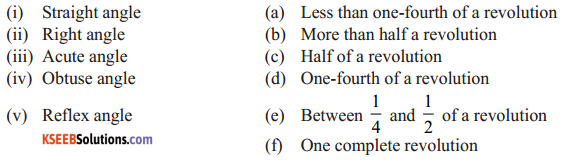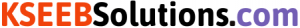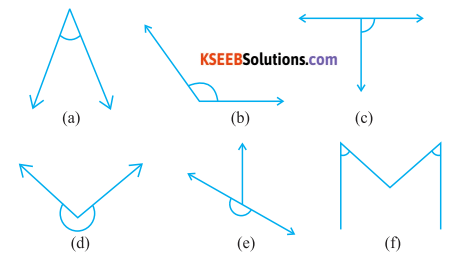# KSEEB Solutions for Class 6 Maths Chapter 5 Understanding Elementary Shapes Ex 5.3

Students can download Chapter 5 Understanding Elementary Shapes Ex 5.3 KSEEB Solutions for Class 6 Maths helps you to revise the complete syllabus.

## Karnataka State Syllabus Class 6 Maths Chapter 5 Understanding Elementary Shapes Ex 5.3

Question 1.
Match the following :Solution:
i) Straight angle is 180° and half of a revolution is 180°. Hence. (i) – (c)
ii) Right angle is 90° and one-fourth of a revolution is 90°. Hence, (ii) – (d)
iii) Acute angles are angles less than 90°. Also, less than one-fourth of a revolution is the angle less than 90°. Hence, (iii) – (a)
iv) Obtuse angles are angles greater than 90° but less than 180°. Also between 1/4 and 1/2 of a revolution is the angle whose measure lies between 90° and 180°. Hence, (iv) – (e)
v) Reflex angles are the angles greater than 180° but less than 180° but less than 360°, Also more than half a revolution is the angle whose measure is greater than 180°.
Hence (V) ⟷ (b)

Use this simple reference angle calculator to find the reference angle of any angle. Learn how to find a reference angle without a calculator.Question 2.
Classify each one of the following angles as right, straight, acute, obtuse, or reflex:Solution:
a) Acute angle as its measure is less than 90°
b) Obtuse angle as its measure is more than 90° but less than 180°
c) Right angle as its measure is 90°
d) Reflex angle as its measure is more than 180° but less than 360°
e) Straight angle as its measure is 180°
f) Acute angle as its measure is less than 90°